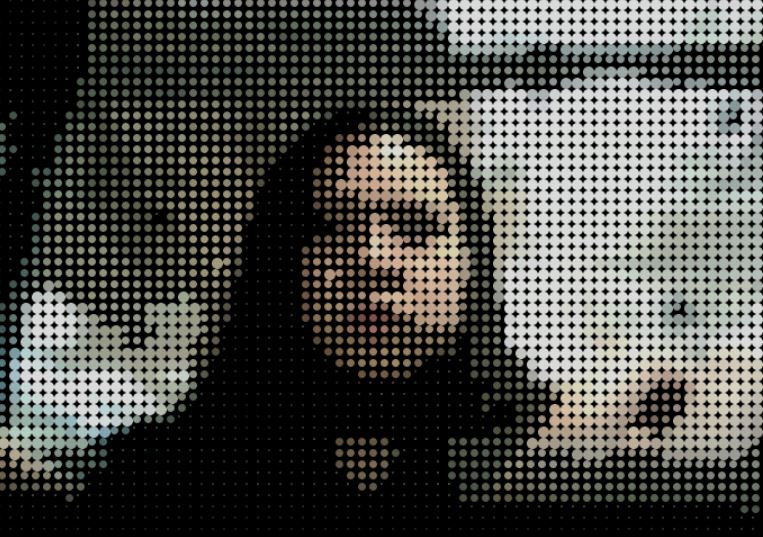Search
• KJ Ha

# Pixel: Interactive Mirror

This sketch was developed from the 1.2 worksheet. I designed the interactive mirror where the brighter pixels have larger sizes. All pixels are represented by different sizes of circles and the brightness of the pixels is an indicator determining the size of the radius.For the pixels whose brightness values are over 66%, they will have a radius of 10 and for those whose values are lower than 66% and higher than 33% will have a radius of 7. Any other pixels whose values are lower than 33% will have a radius of 2.To add some interactive elements into the sketch, I used a map function that is fed by the user's mouse location. Depending on the mouse's x location, the alpha value will change.Where alpha value is close to 0.Where alpha value is close to 255.Another interactive element is associated with a keyTyped function. If the 'r' key is typed, the value of the variable i is changed into 255 making the r value to reach the maximum level (over 255). Therefore, it changes the overall tonality of the sketch into the red. The global variables i, j, and k affect each r, g, b values in the same way (i.e. the 'g' key for green and the 'b' key for blue respectively).When the 'r' key is typed.When the 'g' key is typed.When the 'b' key is typed.

See All